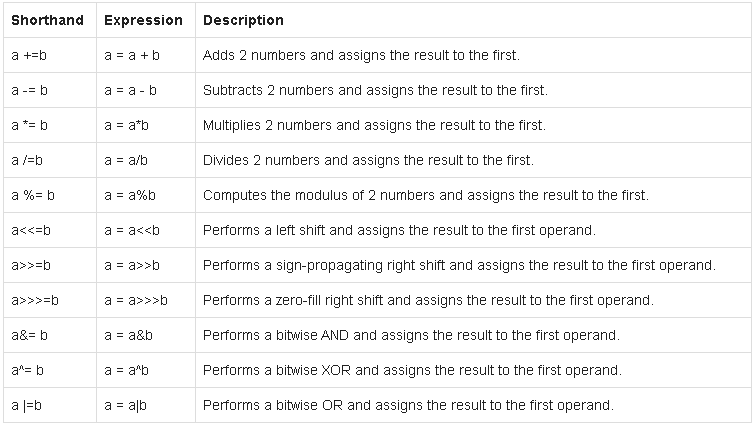# blog posts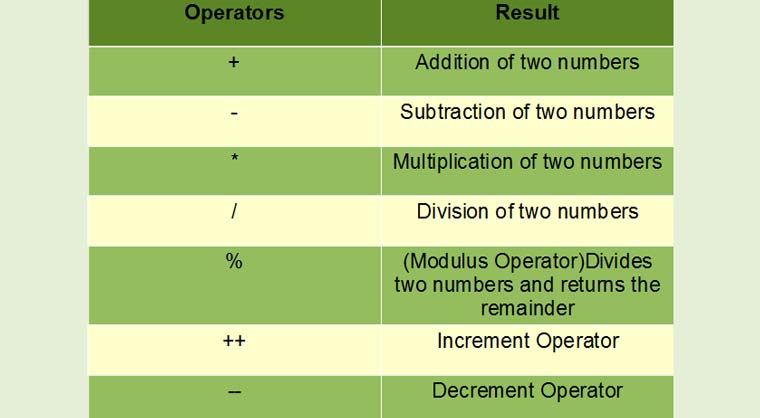# Operators are special symbols (characters) that perform operations on operands (variables and values). For example, the + operator is a plural.

In this tutorial, you will learn everything about the different types of operators in the Java programming language, how to use them by example.

## Assignment Operator

Assignment operators in Java are used to assign values ​​to variables. For example

int age;

age = 5;

The assignment operator assigns its right value to the variable on its left. Here, 5 is assigned to the age variable using the = operator.

There are other assignment operators. However, for convenience, we will train other assignment operators later.

Example 1: Assignment operator

1. class AssignmentOperator {
2. public static void main (String [] args) {
3. int number1, number2;
4. // Assigning 5 to number1
5. number1 = 5;
6. System.out.println (number1);
7. // Assigning value of variable number2 to number1
8. number2 = number1;
9. System.out.println (number2);
10. }
11. }

Output

5

5

## Mathematical Operators

Mathematical operators are used to perform mathematical operations such as addition, subtraction, multiplication, and so on.Example 2: Mathematical operator

1. class ArithmeticOperator {
2. public static void main (String [] args) {
3. double number1 = 12.5, number2 = 3.5, result;
5. result = number1 + number2;
6. System.out.println (“number1 + number2 =” + result);
7. // Using subtraction operator
8. result = number1 – number2;
9. System.out.println (“number1 – number2 =” + result);
10. // Using multiplication operator
11. result = number1 * number2;
12. System.out.println (“number1 * number2 =” + result);
13. // Using division operator
14. result = number1 / number2;
15. System.out.println (“number1 / number2 =” + result);
16. // Using remainder operator
17. result = number1% number2;
18. System.out.println (“number1% number2 =” + result);
19. }
20. }

Output

number1 + number2 = 16.0

number1 – number2 = 9.0

number1 * number2 = 43.75

number1 / number2 = 3.5714285714285716

number1% number2 = 2.0

In the example above, all operands used are variable. Numbers can also be used, they do not have to be variable.

result = number1 + 5.2;

result = 2.3 + 4.5;

number2 = number1 -2.9;

The + operator can also be used to join two or more strings.

Example 3: Mathematical operator

1. class ArithmeticOperator {
2. public static void main (String [] args) {
3. String start, middle, end, result;
4. start = “Talk is cheap. “;
5. middle = “Show me the code. “;
6. end = “- Linus Torvalds”;
7. result = start + middle + end;
8. System.out.println (result);
9. }
10. }

Output

Talk is cheap. Show me the code. – Linus Torvalds

Unit operators

Operations are performed on only one operand.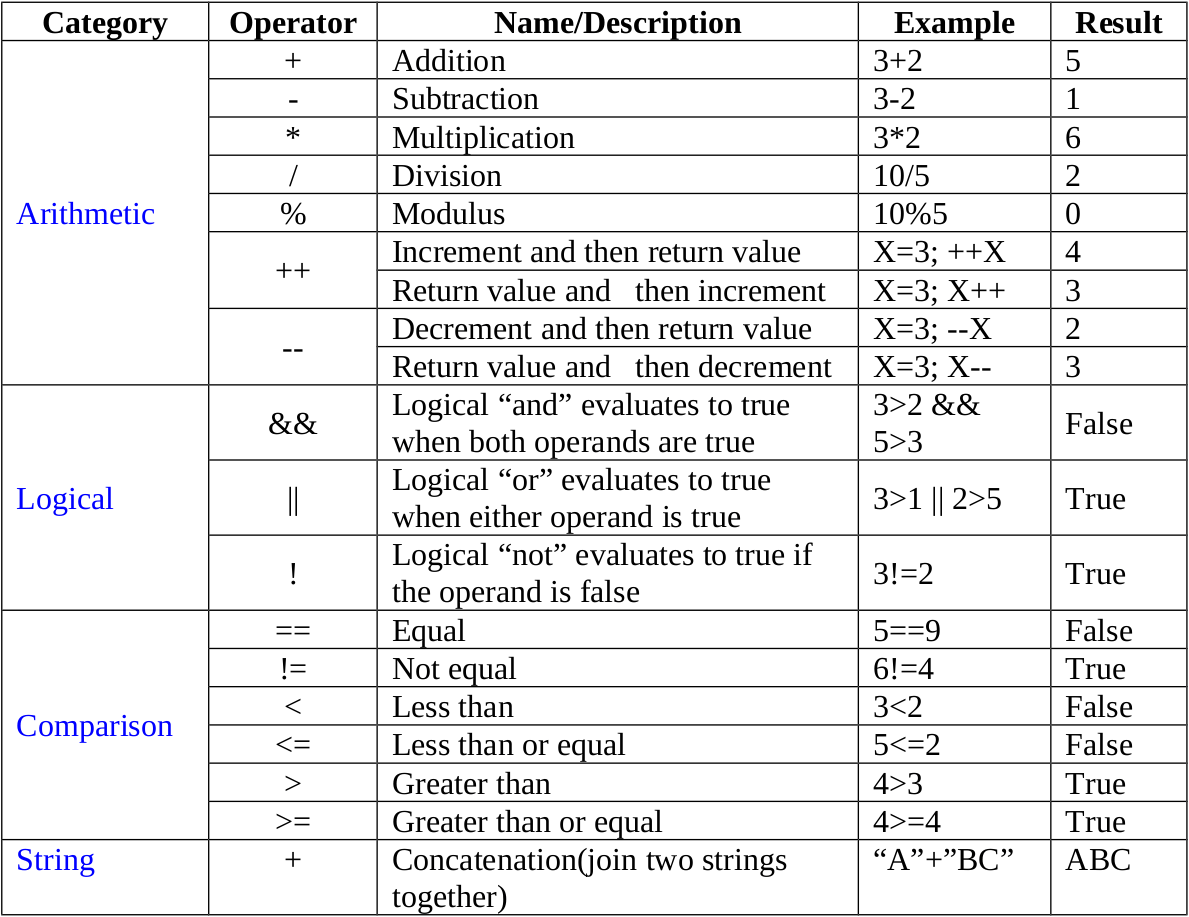Example 4: Unit operator

1. class UnaryOperator {
2. public static void main (String [] args) {
3. double number = 5.2, resultNumber;
4. boolean flag = false;
5. System.out.println (“+ number =” + + number);
6. // number is equal to 5.2 here.
7. System.out.println (“- number =” + -number);
8. // number is equal to 5.2 here.
9. // ++ number is equivalent to number = number + 1
10. System.out.println (“number =” + ++ number);
11. // number is equal to 6.2 here.
12. // – number is equivalent to number = number – 1
13. System.out.println (“number =” + –number);
14. // number is equal to 5.2 here.
15. System.out.println (“! Flag =” +! Flag);
16. // flag is still false.
17. }
18. }

Output

+ number = 5.2

-number = -5.2

number = 6.2

number = 5.2

! flag = true

You can also use ++ and – as prefixes and extensions in Java. The ++ operator increases the value by 1 unit and – decreases the value by 1 unit.

int myInt = 5;

++ myInt // myInt becomes 6

myInt ++ // myInt becomes 7

–MyInt // myInt becomes 6

myInt– // myInt becomes 5

There is a fundamental difference when using incremental and decrement operators as prefixes and suffixes. Consider the following example,

1. class UnaryOperator {
2. public static void main (String [] args) {
3. double number = 5.2;
4. System.out.println (number ++);
5. System.out.println (number);
6. System.out.println (++ number);
7. System.out.println (number);
8. }
9. }

Output

5.2

6.2

7.2

7.2

When ordered

System.out.println (number ++)

Runs, first prints the original value and then increments one unit. That’s why the output is 5.2. Then, when

System.out.println (number)

Runs, prints 6.2.

But, when

System.out.println (++ number)

Runs, before printing on the screen, its value increases by 1 unit.

For – the same is true.

## Equality and relational operators

Equality and relational operators determine the relationship between two operators. Checks that one operand is larger, smaller, equal, unequal, and از larger than another. Depending on the relationship, the result is right or wrong.Equality and relational operators are used in decision making and loops.

Example 6: Equality and relational operators

1. class RelationalOperator {
2. public static void main (String [] args) {
3. int number1 = 5, number2 = 6;
4. if (number1> number2)
5. {
6. System.out.println (“number1 is greater than number2.”);
7. }
8. else
9. {
10. System.out.println (“number2 is greater than number1.”);
11. }
12. }
13. }

Output

number2 is greater than number1.

Here, we used <to check if number1 is greater than number2.

Because number2 is greater than number1, the expression number1> number2 is considered incorrect.

Hence, the else part code is executed and if the condition is true, the code inside the if body is executed.

Remember that equality and relational operators compare two operators and evaluate them as true or false.

In addition to relational operators, there is an example comparative operator that compares an instance of an object with a specific type.

## The instanceof operator

Here is an example of an instanceof operator.

1. class instanceofOperator {
2. public static void main (String [] args) {
3. String test = “asdf”;
4. boolean result;
5. result = instanceof test String;
6. System.out.println (result);
7. }
8. }

When you run the program, the output will be true. This is because test is an example of a String class.

Logical operators

Logical Operators || (Conditional-OR) and && (Conditional-AND) work with Boolean expressions.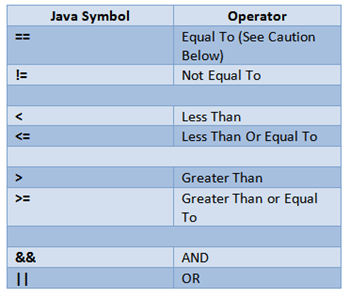Example 8: Logical operator

1. class LogicalOperator {
2. public static void main (String [] args) {
3. int number1 = 1, number2 = 2, number3 = 9;
4. boolean result;
5. // At least one expression needs to be true for result to be true
6. result = (number1> number2) || (number3> number1);
7. // result will be true because (number1> number2) is true
8. System.out.println (result);
9. // All expression must be true from result to be true
10. result = (number1> number2) && (number3> number1);
11. // result will be false because (number3> number1) is false
12. System.out.println (result);
13. }
14. }

Output

true

false

Logical operators are used in decision making and looping.

## Triple operators

Conditional operator or triple operator?: Is an abbreviated if-else structure. The syntax of the conditional operator is:

variable = Expression? expression1: expression2

Here’s how it works.

• If Expression is true, the value of expression1 is assigned to the variable.
• If Expression is false, the value of expression2 is assigned to the variable.

Example 9: Triple operator

1. class ConditionalOperator {
2. public static void main (String [] args) {
3. int februaryDays = 29;
4. String result;
5. result = (februaryDays == 28)? “Not a leap year”: “Leap year”;
6. System.out.println (result);
7. }
8. }

Output

Leap year

## Bitwise and Bit Shift operators

These operators are used to perform bit operations and bit shifts in Java.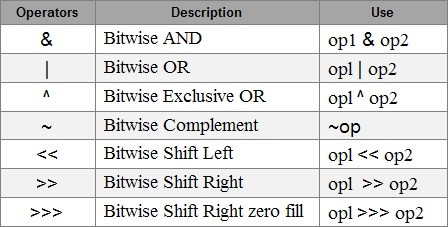These operators are not commonly used.

## Other assignment operators

We only talked about one assignment operator = at the beginning of the tutorial. In addition to this operator, there are other assignment operators that help us write cleaner code.